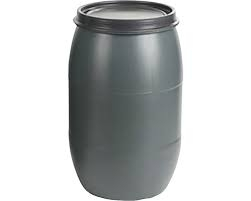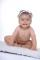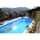# Empty barrel

In the backyard is an empty barrel with a volume of 2 hl. How many liters are missing at the edge, when 53 liters flowed into it for 1 day and 68 liters of water for 2 days?

Correct result:

V3 =  79 l

#### Solution:We would be pleased if you find an error in the word problem, spelling mistakes, or inaccuracies and send it to us. Thank you!Tips to related online calculators
Do you know the volume and unit volume, and want to convert volume units?

## Next similar math problems:

• Cube and waterHow many liters of water can fit into a cube with an edge length of 0.11 m?
• WaterInto a full cylindrical tank high 3 with base radius 2.4 m we insert cuboid with dimensions 1.7 m, 1.7 m, 0.8 m. How many liters of water will come out?
• Water tankIn an empty fire tank, 2150 hl of water jetted in 5 hours. How many hectoliters of water was jetted every hour? How many hectoliters of water was in the tank after three hours?
• The potThe pot is in 1/3 filled with water. Bottom of the pot has an area of ​​329 cm2. How many centimeters rises water level in the pot after add 1.2 liters of water?Grandfather have three barrels each with a capacity 2HL in the garden. How many times he can fill 10L can if two barrels are full and the third is filled with water to the half? How long it lasts water when irrigate the garden 125 liters a day?
• Infants waterFrom the tank, which has 10 hectoliters of water for infants, went 580 liters at mornening and 340 liters in the afternoon. How many liters left in the tank?
• Empty and fullAn empty can has a mass of 1/6 lb. When it is filled with sand, it has a mass of 7/12 lb. Find the mass of the sand in the can?
• Cylindrical tank9.6 hl of water is poured into a cylindrical tank with a bottom diameter of 1.2 m. What height in centimeters does the water reach?
• Three-quarters of its volumeThe pool has a block shape with a length of 8m, a width of 5.3m and a depth of 1.5m. How many hl of water is in it if it is filled to three-quarters of its volume?
• Water tank 2Water tank cuboid is 12 meters long and 6.5 meters wide and 1.2 meters high. How many hectoliters are in the tank when it is filled to 81%?
• Water poolWhat water level is in the pool shaped cuboid with bottom dimensions of 25 m and 10 meters, when is 3750hl water in the pool.
• Hectoliter into cubeCan 1hl of water fit into a half-meter cube?
• IcerinkRectangular rink with dimensions of 68.7 m and 561 dm must be covered with a layer of ice 4.2 cm thick. How many liters of water is necessary for the formation of ice when the volume of ice is 9.7% greater than the volume of water.
• Cylindrical tank 2If a cylindrical tank with volume is used 12320cm raised to the power of 3 and base 28cm is used to store water. How many liters of water can it hold?
• Swimming poolThe swimming pool has the shape of a block with dimensions of 70dm, 25m, 200cm. How many hl of water can fit into the pool?
• AquariumHow many liters of water can fit in an aquarium measuring 30, 15 and 20 cm?
• The tankThe tank has 1320 liters of water. The tank has the shape of a prism, its base is an rectangle with sides a = 0,6 m and b = 1,5 m. How high does the water level reach in the tank?